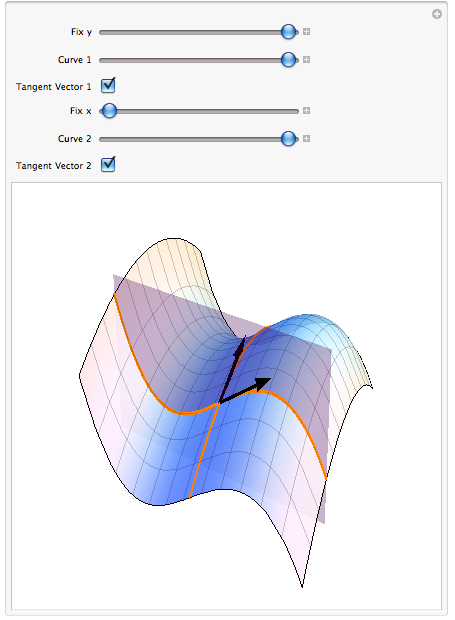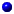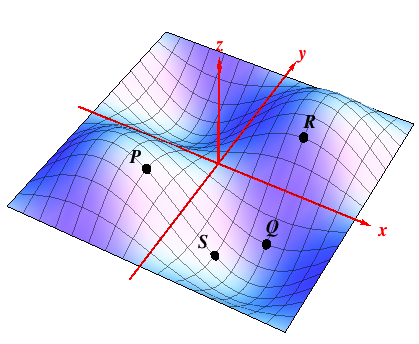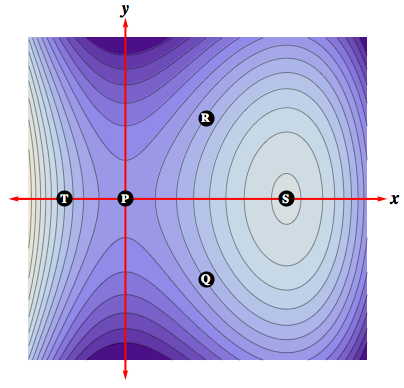Home

#### Integration by Parts

Integration by Parts
Examples
Integration by Parts with a definite integral
Going in Circles

#### Integrals of Trig Functions

Antiderivatives of Basic Trigonometric Functions
Product of Sines and Cosines (mixed even and odd powers or only odd powers)
Product of Sines and Cosines (only even powers)
Product of Secants and Tangents
Other Cases

#### Trig Substitutions

How Trig Substitution Works
Summary of trig substitution options
Examples
Completing the Square

#### Partial Fractions

Introduction to Partial Fractions
Linear Factors
Improper Rational Functions and Long Division
Summary

#### Strategies of Integration

Substitution
Integration by Parts
Trig Integrals
Trig Substitutions
Partial Fractions

#### Improper Integrals

Type 1 - Improper Integrals with Infinite Intervals of Integration
Type 2 - Improper Integrals with Discontinuous Integrands
Comparison Tests for Convergence

#### Modeling with Differential Equations

Introduction
Separable Equations
A Second Order Problem

#### Euler's Method and Direction Fields

Direction Fields
Euler's method revisited

#### Separable Equations

The Simplest Differential Equations
Separable differential equations
Mixing and Dilution

#### Models of Growth

Exponential Growth and Decay
The Zombie Apocalypse (Logistic Growth)

#### Linear Equations

Linear ODEs: Working an Example
The Solution in General
Saving for Retirement

#### Parametrized Curves

Three kinds of functions, three kinds of curves
The Cycloid
Visualizing Parametrized Curves
Tracing Circles and Ellipses
Lissajous Figures

#### Calculus with Parametrized Curves

Video: Slope and Area
Video: Arclength and Surface Area
Summary and Simplifications
Higher Derivatives

#### Polar Coordinates

Definitions of Polar Coordinates
Graphing polar functions
Video: Computing Slopes of Tangent Lines

#### Areas and Lengths of Polar Curves

Area Inside a Polar Curve
Area Between Polar Curves
Arc Length of Polar Curves

#### Conic sections

Slicing a Cone
Ellipses
Hyperbolas
Parabolas and Directrices
Shifting the Center by Completing the Square

#### Conic Sections in Polar Coordinates

Foci and Directrices
Visualizing Eccentricity
Astronomy and Equations in Polar Coordinates

#### Infinite Sequences

Examples of Infinite Sequences
Limit Laws for Sequences
Theorems for and Examples of Computing Limits of Sequences
Monotonic Covergence

#### Infinite Series

Introduction
Geometric Series
Limit Laws for Series
Test for Divergence and Other Theorems
Telescoping Sums

#### Integral Test

Preview of Coming Attractions
The Integral Test
Estimates for the Value of the Series

#### Comparison Tests

The Basic Comparison Test
The Limit Comparison Test

#### Convergence of Series with Negative Terms

Introduction, Alternating Series,and the AS Test
Absolute Convergence
Rearrangements

The Ratio Test
The Root Test
Examples

#### Strategies for testing Series

Strategy to Test Series and a Review of Tests
Examples, Part 1
Examples, Part 2

#### Power Series

Finding the Interval of Convergence
Power Series Centered at $x=a$

#### Representing Functions as Power Series

Functions as Power Series
Derivatives and Integrals of Power Series
Applications and Examples

#### Taylor and Maclaurin Series

The Formula for Taylor Series
Taylor Series for Common Functions
Adding, Multiplying, and Dividing Power Series
Miscellaneous Useful Facts

#### Applications of Taylor Polynomials

Taylor Polynomials
When Functions Are Equal to Their Taylor Series
When a Function Does Not Equal Its Taylor Series
Other Uses of Taylor Polynomials

#### Functions of 2 and 3 variables

Functions of several variables
Limits and continuity

#### Partial Derivatives

One variable at a time (yet again)
Definitions and Examples
An Example from DNA
Geometry of partial derivatives
Higher Derivatives
Differentials and Taylor Expansions

#### Differentiability and the Chain Rule

Differentiability
The First Case of the Chain Rule
Chain Rule, General Case
Video: Worked problems

#### Multiple Integrals

General Setup and Review of 1D Integrals
What is a Double Integral?
Volumes as Double Integrals

#### Iterated Integrals over Rectangles

How To Compute Iterated Integrals
Examples of Iterated Integrals
Fubini's Theorem
Summary and an Important Example

#### Double Integrals over General Regions

Type I and Type II regions
Examples 1-4
Examples 5-7
Swapping the Order of Integration
Area and Volume Revisited

#### Double integrals in polar coordinates

dA = r dr (d theta)
Examples

#### Multiple integrals in physics

Double integrals in physics
Triple integrals in physics

#### Integrals in Probability and Statistics

Single integrals in probability
Double integrals in probability

#### Change of Variables

Review: Change of variables in 1 dimension
Mappings in 2 dimensions
Jacobians
Examples
Bonus: Cylindrical and spherical coordinates

To understand partial derivatives geometrically, we need to interpret the algebraic idea of fixing all but one variable geometrically. This is equivalent to slicing a surface by a plane to produce a curve in space.

 Start with the surface $$z \ = \ f(x,\, y) \ = \ 3x^2 -y^2 -x^3 +2,$$ shown to the right. Then $$f_x \ = \ \frac{\partial f}{\partial x} \ = \ \frac{\partial } {\partial x}\, \bigl( 3x^2 -y^2 -x^3 +2\bigl) \ = \ 6x -3x^2 \,.$$ To understand what this means, fix a value of $y$, say $y=-1$. Equivalently, slice the surface $z=f(x,y)$ with the plane $y=-1$, as in the figure. The cubic curve of intersection is shown in orange and can be given the parametrization $${\bf r}(x)\, = \, \bigl\langle\, x,\, -1,\, f(x,\,-1)\,\bigl\rangle\,=\, \bigl\langle\, x,\, -1,\, 3x^2 -x^3 +1\,\bigl\rangle\,.$$ A vector tangent to this orange curve is $${\bf r}'(x)\ = \ \bigl\langle\, 1,\, 0,\, 6x -3x^2\,\bigl\rangle \ = \ \bigl\langle\, 1,\, 0,\, f_x\,\bigl\rangle\,,$$ Thus $f_x$ gives the slope of the surface $z = f(x,\,y)$ as we move in the $x$-direction. Likewise, if we fix $x= 1$, we see that $f_y$ gives the slope of the other orange curve (obtained by intersecting our surface with the plane $x=1$) as we move in the $y$-direction. ThusAt a point $P=(a,\,b,\, f(a,\,b))$ on the graph of $z = f(x,\, y)$,The value $\displaystyle\frac{\partial f}{\partial x}\Bigl|_{(a,\,b)}$ is the Slope in the $x$-direction,$\displaystyle \Bigl\langle \, 1,\, 0,\, \frac{\partial f}{\partial x}\Bigl|_{a,\,b}\,\Bigl \rangle$ is a Tangent Vector in the $x$-direction, while $$\frac{\partial f}{\partial y}\Bigl|_{(a,\,b)}\, \qquad \hbox{and} \qquad \Bigl\langle \, 0,\, 1,\, \frac{\partial f}{\partial y}\Bigl|_{a,\,b}\,\Bigl \rangle$$ give the slope and a tangent vector at $P$ in the $y$-direction.

 Example 1: Determine whether $f_x ,\ f_y$ are positive, negative, or zero at the points $P,\ Q, \ R,$ and $S$ on the surface to the right. Solution: Starting at $Q$, the surface slopes up for fixed $x$ as $y$ increases, so $f_y\bigl|_{Q} > 0$, while moving slightly in the $x$ direction takes us neither uphill nor downhill, so so $f_x\bigl|_{Q}= 0$. Likewise, $$f_x\bigl|_{R} \,=\,0,\quad f_y\bigl|_{R} \,>\, 0\,, \qquad f_x\bigl|_{P} \,<\, 0,\, \quad f_y\bigl|_{P} \,=\, 0 \,.$$ We leave the signs of $f_x \bigl|_{S}$ and $f_y\bigl|_S$ to you.All the same ideas carry over in exactly the same way to functions $f(x,\,y,\,z)$ of three or more variables - just don't expect lots of pictures!! The partial derivative $f_z$, for instance, is simply the derivative of $f(x,\,y,\,z)$ with respect to $z$, keeping the variables $x$ AND $y$ fixed now.

Information about the partial derivatives of a function $f(x,\,y)$ can be detected also from the contour map of $f$. Indeed, as one knows from using contour maps to learn whether a path on a mountain is going up or down, or how steep it is, so the sign of the partial derivatives of $f(x,\,y)$ and relative size can be read off from the contour map of $f$.

 Example 2: To the right is the contour map of the earlier function $$f(x,\, y) \ = \ 3x^2 -y^2 -x^3 +2\,,$$ with 'higher ground' being shown in lighter colors and 'lower ground' in darker colors. Determine whether $f_x,\, f_y$ are positive, negative, or zero at $P,\, Q,\, R,\, S$, and $T$. At $R$, the colors are lighter (higher) to the right and darker (lower) to the left, so $f_x \bigl |_R > 0$. Likewise, the colors are lighter below $R$ than above $R$, so $f_y \bigl |_R<0$. At $P$ and at $S$, the ground is flat: $S$ is at a local maximum, and $P$ is at a saddle. In both cases, $f_x=f_y=0$. We leave the remaining points to you.